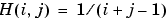MATLAB Function Referencehilb

Hilbert matrix

Syntax

• ```H = hilb(n)
```

Description

```H = hilb(n) ``` returns the Hilbert matrix of order `n`.

Definition

The Hilbert matrix is a notable example of a poorly conditioned matrix . The elements of the Hilbert matrices are.

Examples

Even the fourth-order Hilbert matrix shows signs of poor conditioning.

• ```cond(hilb(4)) =
1.5514e+04
```

 Note    See the M-file for a good example of efficient MATLAB programming where conventional `for` loops are replaced by vectorized statements.

See Also

`invhilb`

References

  Forsythe, G. E. and C. B. Moler, Computer Solution of Linear Algebraic Systems, Prentice-Hall, 1967, Chapter 19.

© 1994-2005 The MathWorks, Inc.